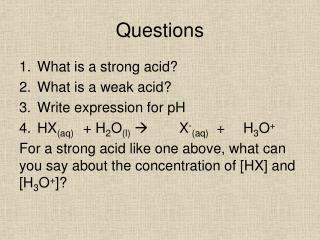DownloadDownload PresentationQuestions

# Questions

Télécharger la présentation## Questions

- - - - - - - - - - - - - - - - - - - - - - - - - - - E N D - - - - - - - - - - - - - - - - - - - - - - - - - - -
##### Presentation Transcript

1. Questions • What is a strong acid? • What is a weak acid? • Write expression for pH • HX(aq) + H2O(l) X-(aq) + H3O+ For a strong acid like one above, what can you say about the concentration of [HX] and [H3O+]?

2. Water dissociation constant, Kw Kw = [H3O+] x [OH-] Increases with increased temperature At 25 deg Kw = 1X10-14

3. Weak Acids Do not fully dissociate so: • Are in equilibrium biased to left • Have a low concentration of ions • Are poor conductors of electricity • React slowly as [H3O+] is low CH3COOH + H2O n CH3COO- + H3O+ As they are in equilibrium, when they are diluted water concentration is increased and there is a shift to the right to compensate. This means predicting pH increase is harder.

4. Acidity Constant, Ka Equilibrium constant Kc = But water is a solvent so Kc = So Ka = [H3O+] [A-] [HA] [H2O] [H3O+] [A-] [HA] [H3O+] [A-] [HA]

5. Weak acid pH Because So Ka = Rearrange and pH = -log[H3O+] Now try Exercise 13C [H3O+] = [A-] [H3O+]2 [HA] [H3O+]= Ka x [HA]

6. pKa pKa= -logKa As the strength of acid increase Ka gets bigger pKagets smaller Conjugate base gets weaker